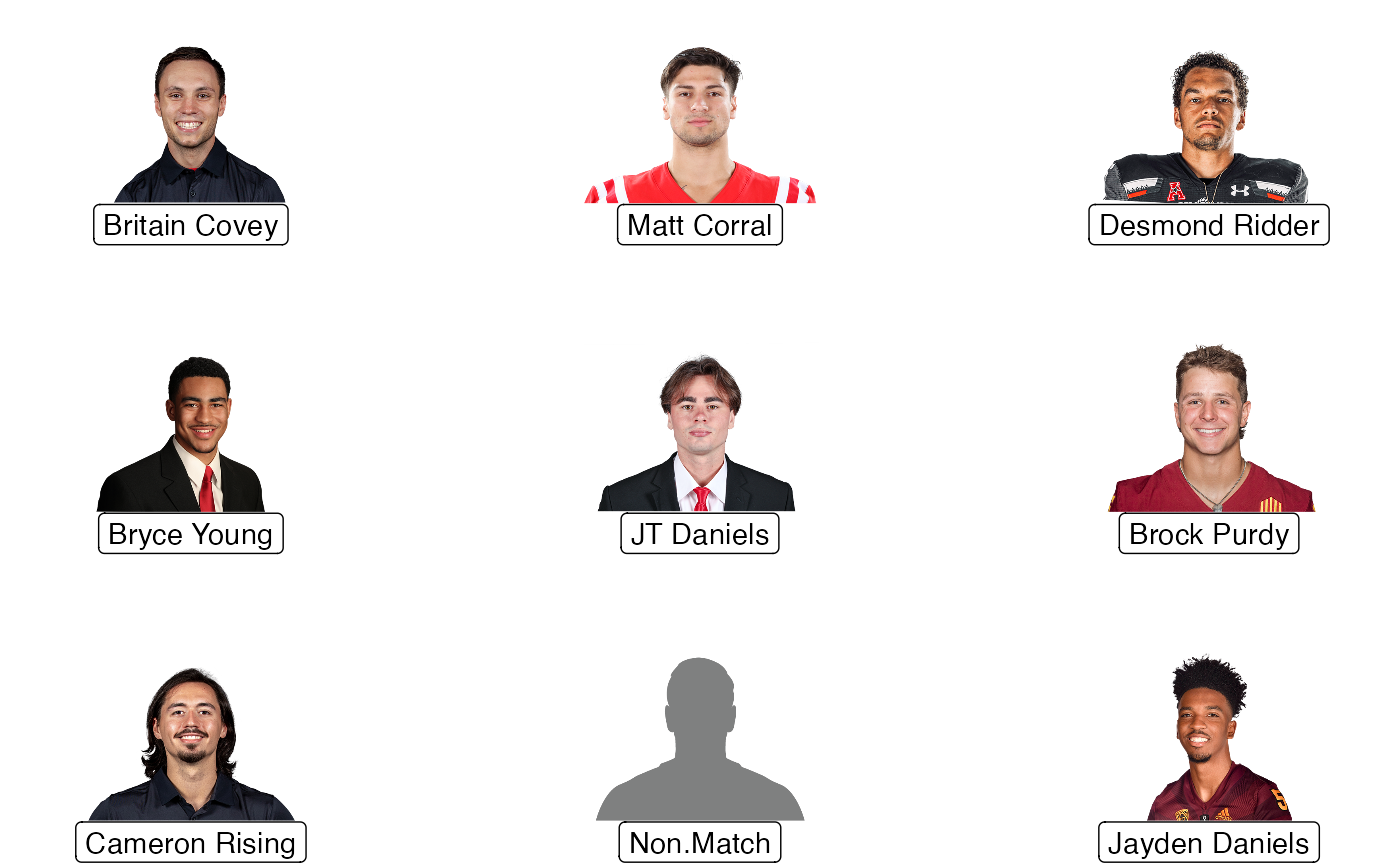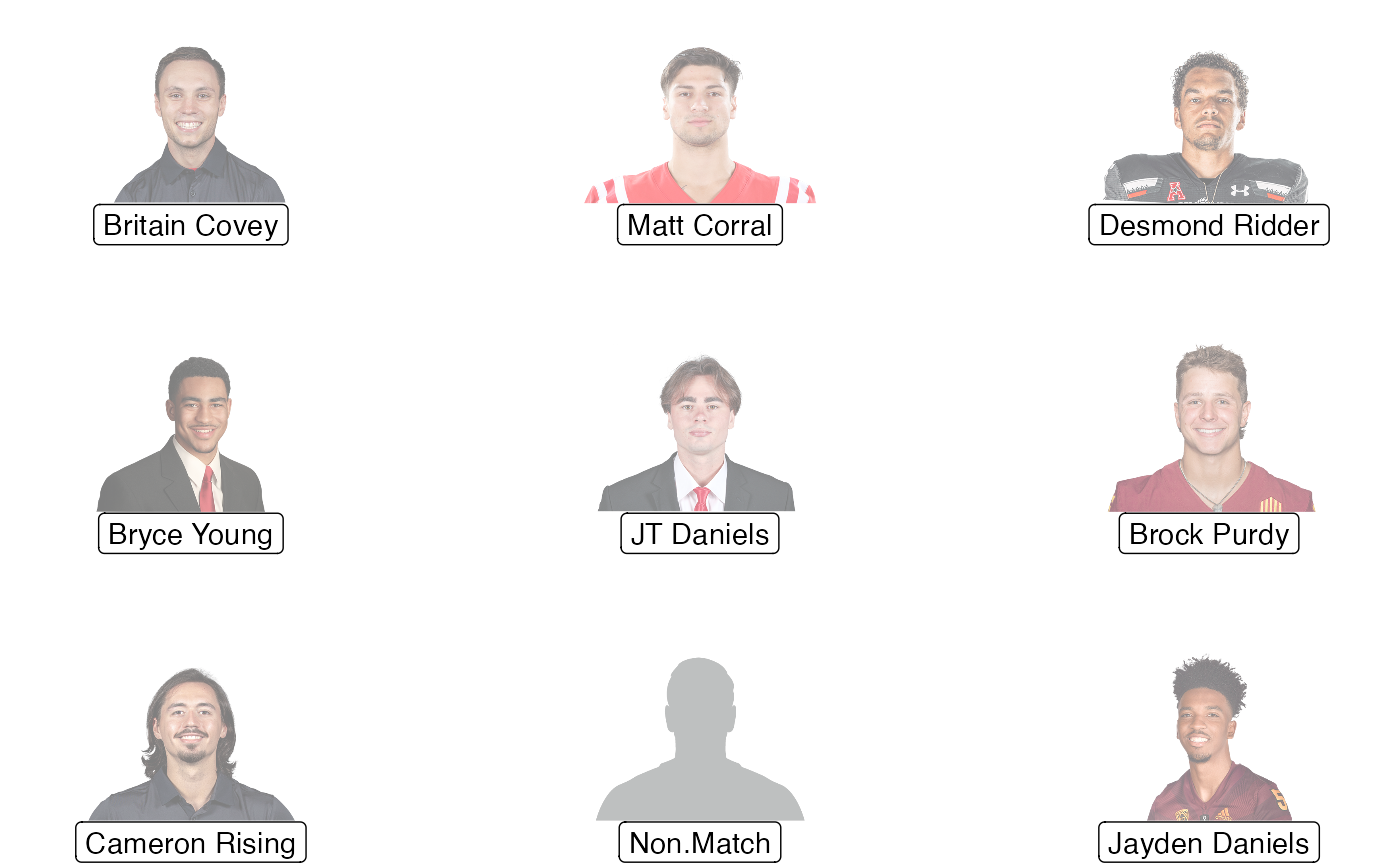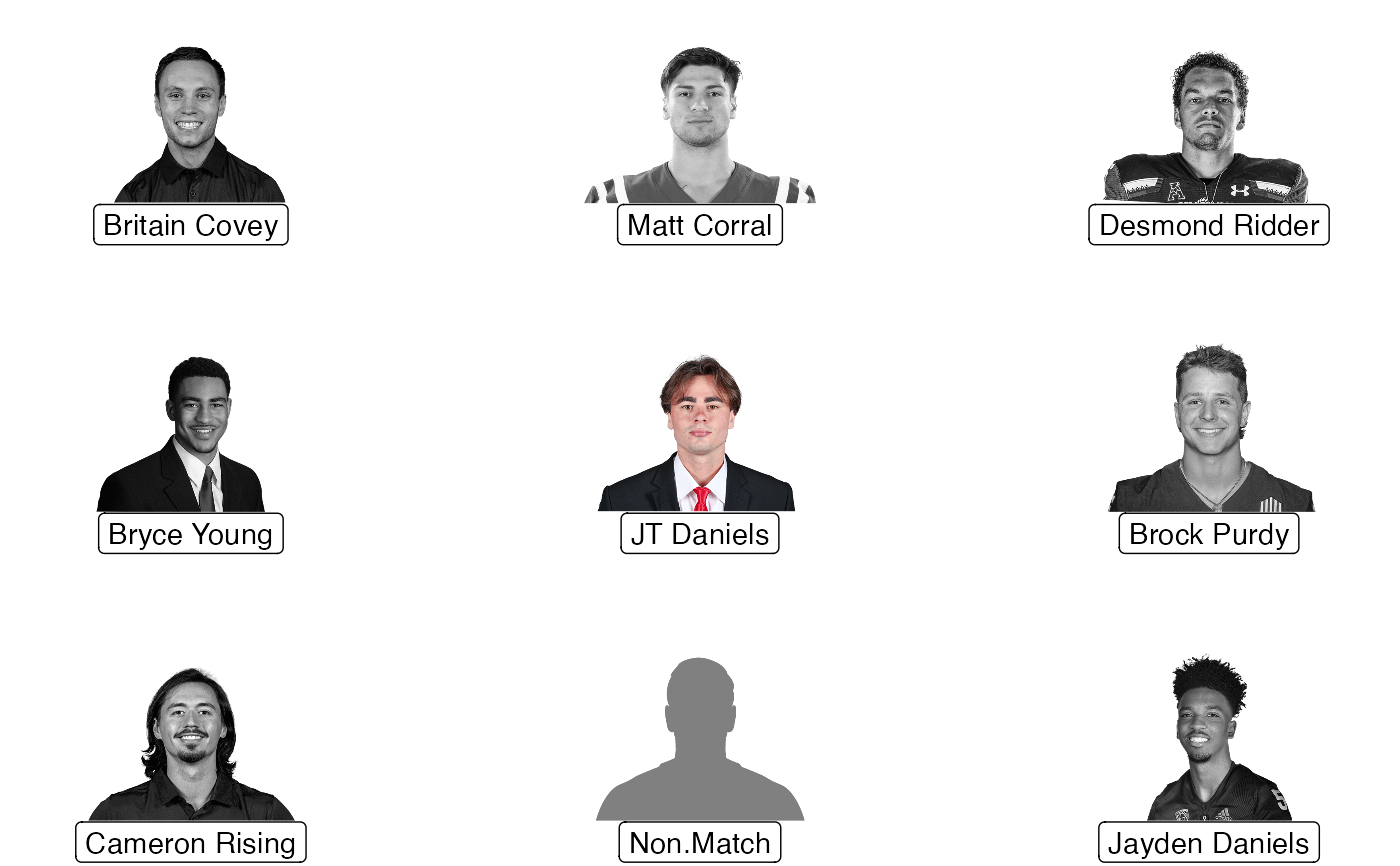This geom is used to plot CFB player headshots instead of points in a ggplot. It requires x, y aesthetics as well as a valid ESPN player id from cfbfastR

geom_cfb_headshots(
mapping = NULL,
data = NULL,
stat = "identity",
position = "identity",
...,
na.rm = FALSE,
show.legend = FALSE,
inherit.aes = TRUE
)

## Arguments

mapping

Set of aesthetic mappings created by aes() or aes_(). If specified and inherit.aes = TRUE (the default), it is combined with the default mapping at the top level of the plot. You must supply mapping if there is no plot mapping.

data

The data to be displayed in this layer. There are three options:

If NULL, the default, the data is inherited from the plot data as specified in the call to ggplot().

A data.frame, or other object, will override the plot data. All objects will be fortified to produce a data frame. See fortify() for which variables will be created.

A function will be called with a single argument, the plot data. The return value must be a data.frame, and will be used as the layer data. A function can be created from a formula (e.g. ~ head(.x, 10)).

stat

The statistical transformation to use on the data for this layer, as a string.

position

Position adjustment, either as a string, or the result of a call to a position adjustment function.

...

Other arguments passed on to ggplot2::layer(). These are often aesthetics, used to set an aesthetic to a fixed value. See the below section "Aesthetics" for a full list of possible arguments.

na.rm

If FALSE, the default, missing values are removed with a warning. If TRUE, missing values are silently removed.

show.legend

logical. Should this layer be included in the legends? NA, the default, includes if any aesthetics are mapped. FALSE never includes, and TRUE always includes. It can also be a named logical vector to finely select the aesthetics to display.

inherit.aes

If FALSE, overrides the default aesthetics, rather than combining with them. This is most useful for helper functions that define both data and aesthetics and shouldn't inherit behaviour from the default plot specification, e.g. borders().

## Aesthetics

geom_cfb_headshots() understands the following aesthetics (required aesthetics are in bold):

• x - The x-coordinate.

• y - The y-coordinate.

• player_id - The players' ESPN id.

• alpha = NULL - The alpha channel, i.e. transparency level, as a numerical value between 0 and 1.

• colour = NULL - The image will be colorized with this colour. Use the special character "b/w" to set it to black and white. For more information on valid colour names in ggplot2 see https://ggplot2.tidyverse.org/articles/ggplot2-specs.html?q=colour#colour-and-fill

• angle = 0 - The angle of the image as a numerical value between 0° and 360°.

• hjust = 0.5 - The horizontal adjustment relative to the given x coordinate. Must be a numerical value between 0 and 1.

• vjust = 0.5 - The vertical adjustment relative to the given y coordinate. Must be a numerical value between 0 and 1.

• width = 1.0 - The desired width of the image in npc (Normalised Parent Coordinates). The default value is set to 1.0 which is big but it is necessary because all used values are computed relative to the default. A typical size is width = 0.075 (see below examples).

• height = 1.0 - The desired height of the image in npc (Normalised Parent Coordinates). The default value is set to 1.0 which is big but it is necessary because all used values are computed relative to the default. A typical size is height = 0.1 (see below examples).

## Examples

# \donttest{
library(cfbplotR)
library(ggplot2)

df <- data.frame(
a = rep(1:3, 3),
b = sort(rep(1:3, 3), decreasing = TRUE),
player_id = c("3926231",
"4362874",
"4239086",
"4685720",
"4374303",
"4361741",
"4362075",
"1",
"4426348"),
player_name = c("Britain Covey",
"Matt Corral",
"Desmond Ridder",
"Bryce Young",
"JT Daniels",
"Brock Purdy",
"Cameron Rising",
"Non.Match",
"Jayden Daniels")
)

# set a custom fill colour for one player
df$colour <- ifelse(df$a == 2 & df\$b == 2, NA, "b/w")

# scatterplot of the headshots
ggplot(df, aes(x = a, y = b)) +
geom_cfb_headshots(aes(player_id = player_id), height = 0.2) +
geom_label(aes(label = player_name), nudge_y = -0.35, alpha = 0.5) +
coord_cartesian(xlim = c(0.75, 3.25), ylim = c(0.7, 3.25)) +
theme_void()
#> Warning: 1 is not a valid player id (row 8)# apply alpha as constant
ggplot(df, aes(x = a, y = b)) +
geom_cfb_headshots(aes(player_id = player_id), height = 0.2, alpha = 0.5) +
geom_label(aes(label = player_name), nudge_y = -0.35, alpha = 0.5) +
coord_cartesian(xlim = c(0.75, 3.25), ylim = c(0.7, 3.25)) +
theme_void()
#> Warning: 1 is not a valid player id (row 8)# apply colour as an aesthetic
ggplot(df, aes(x = a, y = b)) +
geom_cfb_headshots(aes(player_id = player_id, colour = colour), height = 0.2) +
geom_label(aes(label = player_name), nudge_y = -0.35, alpha = 0.5) +
coord_cartesian(xlim = c(0.75, 3.25), ylim = c(0.7, 3.25)) +
scale_colour_identity() +
theme_void()
#> Warning: 1 is not a valid player id (row 8)# }